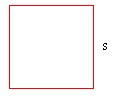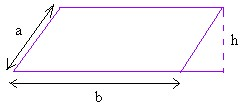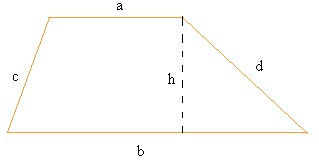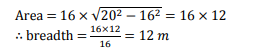# SSC CGL 2020 Study Material: Formulas of Area and Perimeter

SSC CGL 2019-20 is one of the highest demanded competitive examination and scoring good marks in this examination is very challenging. The number of aspirants appearing for this examination is exponentially increasing and making the competition tougher and to successfully qualify this examination you have to brace yourself for long, smart, and dedicated preparation sessions. Keeping the same in mind, we have compiled the formulas for Area and Perimeter of important Geometrical figures in this SSC CGL 2020 Study Material Blog.

Attempt A Free SSC Mock Test Now

### SSC CGL 2020 Study Material – Formulas of Area and Perimeter

 Figure Area Perimeter Circle – := pi × r2 or = (pi × d2) / 4 = 2 × pi × r or = pi × d Square -:= s2 = s + s + s + s = 4 × s Rectangle -:= l × w = l + l + w + w = 2 × l + 2 × w Triangle -:= (b × h) / 2 = a + b + c Parallelogram -:= b × h = a + a + b + b = 2 × a + 2 × b Rhombus -:= b × h = b + b + b + b = 4 × b Trapezoid -:= (a + b) / 2 × h = a + b + c + d

### SSC CGL 2020 Study Material – Sample Questions

#### SSC CGL 2020 Study Material – Question 1

Q) The perimeters of two squares are 40 cm and 32 cm. Find the perimeter of a third square whose area is equal to the difference of the areas of the two squares.

(a) 4 cm

(b) 6 cm

(c) 24 cm

(d) 30 cm

Answer: Option (c) – 24cm

Side of first square = (40/4) cm ⇒ 10 cm;

Side of second square = (32/4) cm ⇒ 8 cm.

Area of third square = [(10)2 – (8)2] cm2 ⇒ (100 – 64) cm2 ⇒ 36 cm2.

Side of the third square ⇒ 36 cm ⇒ 6 cm.

So, Required perimeter = (6 × 4) cm ⇒ 24 cm.

Attempt SSC CGL Free Mock Test Here

#### SSC CGL 2020 Study Material – Question 2

Q) A man walked 20 m to cross a rectangular field diagonally. If the length of the field is 16 m, the breadth of the field is:

(a) 4 m

(b) 16 m

(c) 12 m

(d) Can’t be determined

Answer = Option (c) – 12 mAttempt SSC CGL Free Mock Test Here

#### SSC CGL 2020 Study Material – Question 3

Q) A copper wire is bent in the form of a square with an area of 121 cm. If the same wire is bent in the form of a circle, the radius (in cm) of the circle is (Take π = 22/7)

(a) 7 cm

(b) 10 cm

(c) 11 cm

(d) 14 cm

Answer – Option (a) – 7cm

Side of square = 121 = 11 cm

∴     Length of wire = 4 × 11 = 44 cm

∴    2πr = 44

⇒   2 × 22/7       × r = 44

⇒   r =   44 × 7/2 × 22 = 7 cm

Attempt SSC CGL Free Mock Test Here

#### SSC CGL 2020 Study Material – Question 4

Q) The width of a rectangular hall is 3/4th of its length. If the area of the hall is 300 sq.m, then the difference between its length and width is:

(a) 3 m

(b) 4 m

(c) 5 m

(d) 15 m

Answer = Option (c) – 5 m#### SSC CGL 2020 Study Material – Question 5

Q) The lengths of two parallel sides of a trapezium are 6 cm and 8 cm. If the height of the trapezium be 4 cm, then its area is.

(a) 28 sq.cm

(b) 30 sq.cm

(c) 32 sq.cm

(d) 26 sq.cm

Answer = Option (a) – 28sq.cm

Area of trapezium = 1/2 × (Sum of parallel sides) × (Perpendicular distance)

=             1/2(6 + 8) × 4

=             1/2 × 14 × 4 = 28 sq. cm

We hope the SSC CGL Study Material 2020 provided in this blog is helpful for your SSC CGL 2020 Exam Preparation.

The following features will be provided in our SSC CGL Mock Tests Series:

Also, Check: